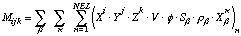# Manual Page for Command >> MOMENT

Syntax
>> MOMENT (FIRST/SECOND) (X/Y/Z) (comp_name/COMPONENT: icomp) (phase_name/PHASE: iphase) (CHANGE/DELTA)

Parent Command
> OBSERVATION

Subcommand
>>> MODEL

Description
This command selects as an observation type the first or second spatial moment in X, Y, or Z direction of component icomp or phase iphase. This observation type refers to all elements with SPHT < 10000. The X, Y, and Z coordinates of the grid blocks must be given in TOUGH2 block ELEME, columns 51-60, 61-70, 71-80, respectively. Component number icomp or component name comp_name, and phase number iphase or phase name phase_name depend on the EOS module being used. They are listed in the iTOUGH2 header, and can be specified either on the command line or using the two subcommands >>>> COMPONENT and >>>> PHASE, respectively. If only a phase but no component is specified, the spatial moment of the indicated phase including all components is calculated. If only a component but no phase is given, the spatial moment of that component in all phases is calculated. If both a component and a phase are given, the spatial moment of the component in the specified phase is calculated.

The spatial moments are calculated as follows:where the first sum is taken over the selected phase(s) beta, the second sum is taken over the selected component(s) kappa, and the third summation accumulates the masses of component kappa in phase beta from all elements that are included in the global mass balance calculation (i.e., elements with a rock grain specific heat lower than 104 J/kg C). The mass in element n is the product of the element volume V, the porosity phi, the saturation S, the phase density rho, and the mass fraction X. The first moment represents the center of mass coordinates which are given by:

<X> = M100/M000<Y> = M010/M000

<Z> = M001/M000

The second moment represents the variances in the three directions given by:

sigma2 = M200/M000 – <X>2sigma2 = M020/M000 – <Y>2

sigma2 = M002/M000 – <Z>2

This option may be especially useful for characterizing the location and spreading of a contaminant or saturation plume. Note that boundary effects may have a strong impact on spatial moment calculation.

Example
> OBSERVATION
>> FIRST MOMENT (Z-coordinate) of TRACER
>>> entire MODEL
>>>> ANNOTATION: plume Z coord.
>>>> NO DATA
<<<<
<<<

>> SECOND MOMENT (X-coordinate) of TRACER
>>> entire MODEL (except boundary elements with SPHT .gt. 10000)